# NCERT Solutions Class 10 Science Chapter 2 Acids Bases And Salts

Ncert Solutions for class 10 subject Science Chapter 2 Acids Bases And Saltsin pdf Best Free NCERT Solutions for class 1 to 12 in pdf NCERT Solutions, cbse board, Science, ncert Solutions for Class 10 Science, class 10 Science ncert solutions, Acids Bases And Salts, Class 10, ncert solutions chapter 2 Acids Bases And Salts, class 10 Science, class 10 Science ncert solutions, Science ncert solutions class 10, Ncert Solutions Class 10 Science Chapter 2 Acids Bases And Salts

## Concept Chapter 2 Class 10 Science Acids Bases And Salts Ncert Solutions

< style="color:#002060">

Concepts: Acids, Bases and Salts

Acids: Those substances which when dissolved in water then produce H+ (aq) or Hydronium (H3O+) ions. They are sour in taste and change the colour of blue litmus to red. For example HCl, H2SO4, HNO3 etc.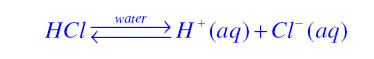Bases: Bases are generally metallic oxide or metallic hydroxide. When they are dissolved in water they produce OH-(aq) ions. They are bitter in taste and change the colour of red litmus to blue. For example CuO, NaOH, KOH etc.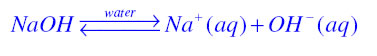Acid-Base Indicators: Indicators are basically dyes or mixtures which are used to detect whether the substance is acidic or basic with the help of their colour changing property. They are of two type, natural indicators and synthetic indicators. The examples of natural indicators are Litmus and turmeric. The examples of synthetic indicators are methyl orange and phenolphthalein.

Olfactory indicators: Those substances whose odour changes in acidic or basic medium are known as olfactory indicators. Some of the examples of olfactory indicators are vanilla, onion and clove.

pH scale: It is the measure of acidic or basic strength of an aqueous solution. The pH value of an acidic solution lies between 0 to 7. The pH value of a basic solution lies between 7 to 14. The pH value of a neutral substance is always 7.

Reaction of acid with a metal: When an acid reacts with a metal then Hydrogen gas is evolved along with corresponding salt.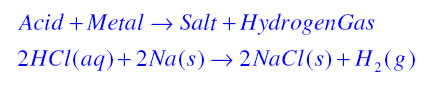Reaction of base with a metal: When a base reacts with a metal then Hydrogen gas is evolved along with corresponding salt. Salt is composed of metal and oxygen and has negative ion.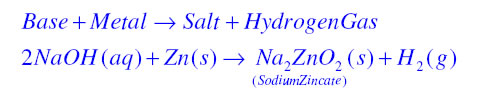How do metal carbonates and Metal Hydrogencarbonates React with Acids?
When Metal carbonates and Metal Hydrogencarbonates react with acid they form corresponding salt with carbon dioxide and water as shown in following chemical equation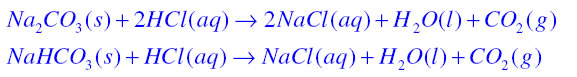Reaction of acids and bases together: Whenever acids and bases react with each other they produce salt with water. For example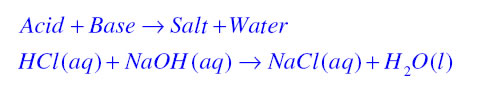Neutralisation reaction: When acid and base react with each other to produce salt and water then such reaction is known as neutralisation reaction. For example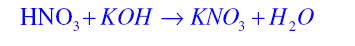Reaction of metallic oxide with acid: When metallic oxide reacts with acid they produce salt along with water. For example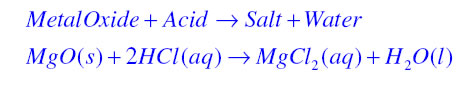Why Acidic and basic solutions conduct electricity?: Acidic and basic solutions in water conduct electricity because they produce hydrogen and hydroxide ions respectively.

Water of crystallisation: It is the definite number of molecules of water that is chemically attached to each formula unit of a salt in its crystalline form. For example washing soda (Na2CO3.10H2O) or Gypsum (CaSO4.2H2O).

Some very important salts with their chemical name and formula.

 Common Name Chemical Name Chemical Formula Baking Soda Sodium Bicarbonate NaHCO3 Washing Soda Hydrated Sodium Carbonate Na2CO3.10H2O Plaster of paris Calcium Sulphate hemihydrates CaSO4.½H2O Bleaching Powder Calcium Oxychloride CaOCl2 Gypsum Calcium Sulphate dihydrate CaSO4.2H2O

## In Text Question Chapter 2 Class 10 Science Acids Bases And Salts Ncert Solutions

In text Questions page number 18

Question 1. You have been provided with three test tubes. One of them contains distilled water and the other two contain an acidic solution and a basic solution, respectively. If you are given only red litmus paper, how will you identify the contents of each test tube?

Answer: If the colour of red litmus does not change then it is acid. If the colour of red litmus changes to blue then it is base. If there is slight change in the colour of red litmus (such as purple) then it is distilled water.

In text Questions page number 22

Question 1. Why should curd and sour substances not be kept in brass and copper vessels?
Answer: Curd and sour substances should not be kept in brass and copper as curd and sour substances are acidic in nature and react with the metallic surface of the brass and copper to form some toxic compound which may be unfit for health.

Question 2. Which gas is usually liberated when an acid reacts with a metal? Illustrate with an example. How will you test for the presence of this gas?
When acids react with metal generally hydrogen gas is produced. We can test it by bringing burning candle near to it then it will produce pop sound.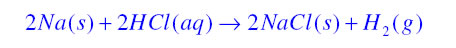Question 3. Metal compound A reacts with dilute hydrochloric acid to produce effervescence. The gas evolved extinguishes a burning candle. Write a balanced chemical equation for the reaction if one of the compounds formed is calcium chloride.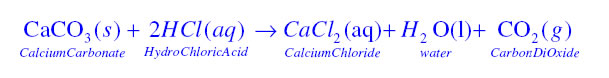In text Questions page number 25

Question 1. Why do HCl, HNO3, etc., show acidic characters in aqueous solutions while solutions of compounds like alcohol and glucose do not show acidic character?
When HCl or HNO3 are mixed with water then they dissolve in water to form H+ or H3O+ ions which shows their acidic character. For example just see the following reactions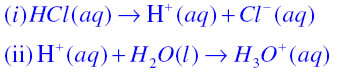When alcohols and glucose are mixed with water then they do not dissolve to form ions. Hence they do not show acidic character.

Question 2. Why does an aqueous solution of an acid conduct electricity?
The presence of hydrogen (H+) or hydronium (H3O+) ions in the aqueous solution of an acid are responsible for conducting electricity.

Question 3. Why does dry HCl gas not change the colour of the dry litmus paper?
The colour of litmus paper changes only in the presence of ions like hydrogen (H+) or hydronium (H3O+) ions. HCl can produce these ions only in the form of aqueous solution. Hence dry HCl gas does not change the colour of dry litmus paper.

Question 4. While diluting an acid, why is it recommended that the acid should be added to water and not water to the acid?
The process of adding water to an acid is highly exothermic. If it is not done in the proper way then may the mixture splash out and causes serious burns. Therefore it is recommended that acid should be added to water carefully.

Question 5. How is the concentration of hydronium ions (H3O+) affected when a solution of an acid is diluted?
When the solution of an acid is diluted then its strength decreases as there is decrease in the concentration of hydronium (H3O+) ions.

Question 6. How is the concentration of hydroxide ions (OH-) affected when excess base is dissolved in a solution of sodium hydroxide?
When excess base is dissolved in the solution of sodium hydroxide then the concentration of hydroxide (OH-) ions increases.

In text Questions page number 28

Question 1. You have two solutions, A and B. The pH of solution A is 6 and pH of solution B is 8. Which solution has more hydrogen ion concentration? Which of this is acidic and which one is basic?
The pH value of a solution varies from 0 to 14. The pH value is 0 for a very strong acid and the pH value is 14 for a very strong base. The pH value is 7 for a neutral solution. Hence A is acidic and B is basic in nature. The concentration of hydrogen ion decreases from pH value of 0 to 14 therefore A has more hydrogen ion concentration.

Question 2. What effect does the concentration of H+(aq) ions have on the nature of the solution?
If the concentration of H+(aq) ions is increased(>10-7) then the solution become acidic and if the concentration of H+(aq) ions is decreased(<10-7) then the solution become basic in nature.

Question 3. Do basic solutions also have H+(aq) ions ? If yes, then why are these basic?
Yes the basic solution also has H+(aq) ions but it is less than the concentration of (OH-)ions.

Question 4. Under what soil condition do you think a farmer would treat the soil of his fields with quick lime (calcium oxide) or slaked lime (calcium hydroxide) or chalk (calcium carbonate)?
If the soil is acidic in nature which is not suitable for agriculture then the farmer would treat the soil with quick lime (calcium oxide) or slaked lime (calcium hydroxide) or chalk (calcium carbonate).

In text Questions page number 33

Question 1. What is the common name of the compound CaOCl2?
Bleaching Powder.

Question 2. Name the substance which on treatment with chlorine yields bleaching powder.
When calcium hydroxide is treated with chlorine gas it yields bleaching powder. It is shown in the following chemical reaction.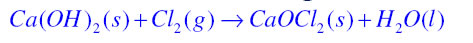Question 3. Name the sodium compound which is used for softening hard water.
Washing Soda (Na2CO3.10H2O) is hydrated sodium carbonate which is used for softening hard water.

Question 4. What will happen if a solution of sodium hydrocarbonate is heated? Give the equation of the reaction involved.
When sodium hydrogen carbonate is heated then sodium carbonate and water is formed along with the evolution of carbon dioxide gas.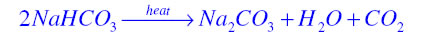Question 5. Write an equation to show the reaction between Plaster of Paris and water.
When plaster of paris reacts with water it produce gypsum as shown in the following chemical reaction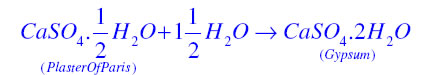## Exercise Chapter 2 Class 10 Science Acids Bases And Salts Ncert Solutions

Text Book Exercise

Question 1. A solution turns red litmus blue; its pH is likely to be
(a) 1 (b) 4 (c) 5 (d) 10
(d)

Question 2. A solution reacts with crushed egg-shells to give a gas that turns lime-water milky. The solution contains
(a) NaCl (b) HCl (c) LiCl (d) KCl
(b)

Question 3. 10 mL of a solution of NaOH is found to be completely neutralised by 8 mL of a given solution of HCl. If we take 20 mL of the same solution of NaOH, the amount HCl solution (the same solution as before) required to neutralise it will be
(a) 4 mL (b) 8 mL (c) 12 mL (d) 16 mL
(d)

Question 4. Which one of the following types of medicines is used for treating indigestion?
(a) Antibiotic
(b) Analgesic
(c) Antacid
(d) Antiseptic
(c)

Question 5. Write word equations and then balanced equations for the reaction taking place when –
(a) dilute sulphuric acid reacts with zinc granules.
(b) dilute hydrochloric acid reacts with magnesium ribbon.
(c) dilute sulphuric acid reacts with aluminium powder.
(d) dilute hydrochloric acid reacts with iron filings.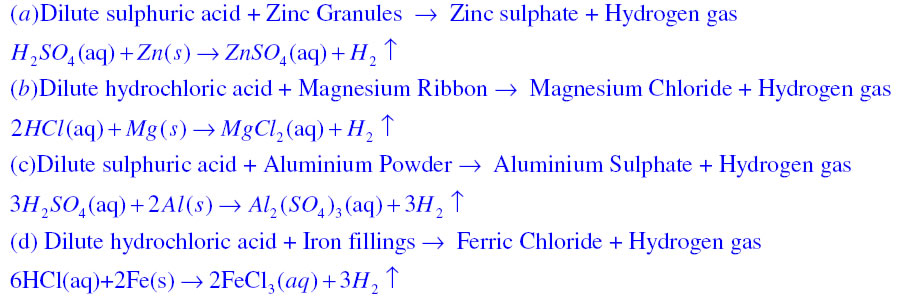Question 6. Compounds such as alcohols and glucose also contain hydrogen but are not categorised as acids. Describe an Activity to prove it.
As shown in figure insert two nails on the wooden or rubber cork and place it in a beaker. Now connect these iron nails with a bulb, a 6 volt battery and a switch using a wire. Now pour some alcohol or glucose such that the nails will dip into it. Now turn the switch on, you will see that the bulb will not glow. Now empty the beaker and add some HCl aqueous solution at this time the bulb will glow. This proves that an acid can conduct electricity while alcohols and glucose cannot, even when they are containing hydrogen.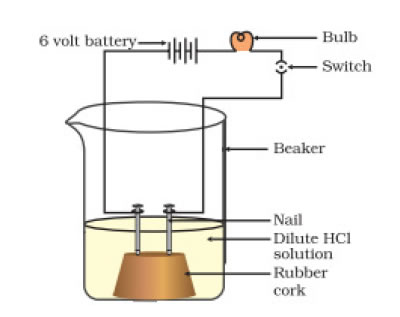Above diagram shows that an acid solution can conduct electricity.

Question 7. Why does distilled water not conduct electricity, whereas rain water does?
Distilled water cannot conduct electricity because it does not contain ions while rain water conducts electricity as it contains ions due presence of dissolved salts in it.

Question 8. Why do acids not show acidic behaviour in the absence of water?
The acidic behaviour of a substance is due to the presence of H+(aq) ions. As acids do not dissociate to produce H+(aq) ions in the absence of water so they do not show acidic behaviour.

Question 9. Five solutions A, B, C, D and E when tested with universal indicator showed pH as 4, 1, 11, 7 and 9, respectively. Which solution is
(a) neutral?
(b) strongly alkaline?
(c) strongly acidic?
(d) weakly acidic?
(e) weakly alkaline?
Arrange the pH in increasing order of hydrogen-ion concentration.
(a) neutral -> D
(b) strongly alkaline -> C
(c) strongly acidic -> B
(d) weakly acidic -> A
(e) weakly alkaline -> E
The arrangement of pH in increasing order of hydrogen-ion concentration is 11<9<7<4<1

Question 10. Equal lengths of magnesium ribbons are taken in test tubes A and B. Hydrochloric acid (HCl) is added to test tube A, while acetic acid (CH3COOH) is added to test tube B. In which test tube will the fizzing occur more vigorously and why?
In both the test tubes hydrogen gas is formed. The HCl is strong acid then acetic acid so more hydrogen gas is formed in test tube A which results in vigorous fizzing.

Question 11. Fresh milk has a pH of 6. How do you think the pH will change as it turns into curd? Explain your answer.
When milk is turned into curd then its pH value will decrease due to the production of lactic acid in curd which is acidic in nature.

Question 12. A milkman adds a very small amount of baking soda to fresh milk.
(a) Why does he shift the pH of the fresh milk from 6 to slightly alkaline?
(b) Why does this milk take a long time to set as curd?

He shifts the pH of the fresh milk from 6 to alkaline so that in basic form it will not spoil easily.

This milk takes a long time to set as curd because the lactic acid produced reacts with the baking soda.

Question 13. Plaster of Paris should be stored in a moisture-proof container. Explain why?
The Plaster of Paris should be stored in a moisture-proof container as it absorbs water from moisture and turn into hard substance (Gypsum) as shown in following chemical equation.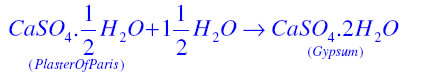Question 14. What is a neutralisation reaction? Give two examples.
When acid and base react with each other to produce salt and water then such reaction is known as neutralisation reaction. For example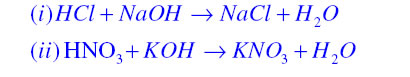Question 15. Give two important uses of washing soda and baking soda.

(a) Two important uses of washing soda

(i) It is used in the manufacture of soap and glass.

(ii) It is used to remove the permanent hardness of water.

(b) Two important uses of baking soda

(i) It is used for making baking powder.

(ii) It is used in soda- fire extinguishers.

### NCERT Books Free Pdf Download for Class 5, 6, 7, 8, 9, 10 , 11, 12 Hindi and English Medium

 Mathematics Biology Psychology Chemistry English Economics Sociology Hindi Business Studies Geography Science Political Science Statistics Physics Accountancy